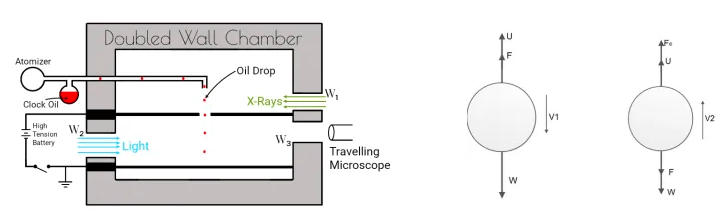# Millikan’s Oil Drop Experiment- Class 12 Physics, NEB

09/16/2020, Nepal.

Electron is a negatively charged common particle found in all types of matter. The term “electron” was first suggested by Stoney in 1891, although the electron was not discovered until 1897, by British physicist J.J. Thomson. Each electron carries one unit of negative charge (1.602 x 10-19 coulomb) and has a very small mass as compared with that of a neutron or proton. The mass of an electron is 9.10938 x 10-31 kg. This is about 1/1836 the mass of a proton. A common symbol for an electron is e.

The electron’s antiparticle, which carries a positive electric charge, is called a positron or antielectron. A positron is denoted using the symbol e+ or β+.

### Properties of the electron:

• Electrons are negatively charged tiny particles.
• The mass of an electron is 9.10938 x 10-31 kg. This is about 1/1836 the mass of a proton.
• They show both particle-like and wave-like behavior.
• Quantum mechanical properties of electron include an intrinsic angular momentum spin of the value of half of reduced Planck's constant.

## Millikan’s oil drop experiment:

Q. Describe the necessary theory and calculations of Millikan’s oil drop experiment for the measurement of the charge of an electron .

The experimental arrangement of Millikan’s oil drop experiment consists of two metal circular plates of about 20cm in diameter and 1.5 cm of separation with a small hole in the center of the upper plate through which a drop of non-volatile oil is sprayed with the help of an atomizer. These oil drops get charged due to friction and get ionized due to X-ray provided through window W1 Also, window W2 is open to provide enough light to illuminate the oil drop as shown in the figure below.These two parallel horizontal plates are connected to two terminals of the H.T battery to provide electric field E as given below in the figure.[a]                                                                            [b]                                   [c]

Fig: Experimental set-up.                                                          Effect of gravity alone.               Gravity+ electric field.

### The experiment is done in the following two steps:

1.The motion of oil drop in the absence of electric field  [Motion of drop under the gravity alone].

In this case, the oil drop falls under the action of gravity alone reaching a constant velocity called terminal velocity when the viscous force becomes equal to its resultant weight. At, terminal velocity, the net force acting on the drop must be zero.

Net Force = 0

i.e, Upthrust + Viscous force = Weight  [fig ‘a’]

U + F = W

Assuming oil drop spherical, Upthrust (U) = volume of air displaced x density of air x acceleration due to gravity.

#### U= \frac {4}{3} \pi r^3 \sigma g

Now, From Stoke’s law, Viscous force is given by;

#### (F)= 6 \pi \eta r v_1

Also, we know, The volume of oil drop = Volume of air displaced.

#### So\; Weight \; of\; oil\; drop\; (W)=\frac {4}{3} \pi r^3 \rho g

where $\rho$ is the density of an oil.

Hence; above equation becomes,

#### \therefore r= \sqrt{\frac{9}{2} \frac{\eta v_1} {(\rho -\sigma) g}}

This equation gives the radius of the oil drop considering the effect of gravity alone.

2.The motion of the oil drop under the combined effect of gravity and electric field:

In this case, the experiment is conducted under the effect of gravity as well as the electric field (E) with total charge ‘q’. When the strength of the electric field is strong enough to cause the oil drop to move in an upward direction with velocity v2 as shown in figure (c) above.

Then, the net force acting on an oil drop = 0.

Here, in this situation, the forces acting in an upward direction are upthrust (U) and electric field (E) whereas viscous force and the weight of the oil drop are acting in a downward direction.

i.e,  Electrostatic force + upthrust = Viscous force + Weight

So, finally ;

#### \therefore q= \frac{6\pi \eta \:(v_1+ v_2)\:r} {E}\\

Using the value of the radius of oil drop (r), the above equation takes the following form;

#### q= 6\pi\eta  \frac{(v_1+v_2)}{E} \big[ \sqrt{\frac{9}{2} \frac{\eta v_1} {(\rho -\sigma) g}}\big]

————[A]

If the oil drop moves in a downward direction even if the electric field is applied, then the above equation modifies as;

#### q= 6\pi\eta  \frac{(v_1-v_2)}{E} \big[ \sqrt{\frac{9}{2} \frac{\eta v_1} {(\rho -\sigma) g}}\big]

————[B]

In this way, we can calculate the total charge carried by an oil drop. By performing this process in large number of time, it is found that the value of ‘q’ is an integral multiple of a small unit of charge equivalent to a charge carried by an electron i.e 1.602 x 10-19 coulomb.

This means that the total charge 'q' on the oil drop can be expressed as;

#### q=ne, \; \; where \; n=1,2,3,.....

Hence, this experiment concludes that the charge on any object exists as a multiple of small units called the quantum of charge. Each quantum of charge is equivalent to 1.602 x 10-19 C. This is called quantization of charge.### Importance of Millikan’s Experiment:

• It shows that electronic charge was the smallest possible charge on a charged particle or ion.
• It proved the quantization of charge i.e body can carry an integral multiple of minimum charge (e).
• It helps to estimate the mass of an electron from the use of the value of the specific charge of an electron.

Watch this video: